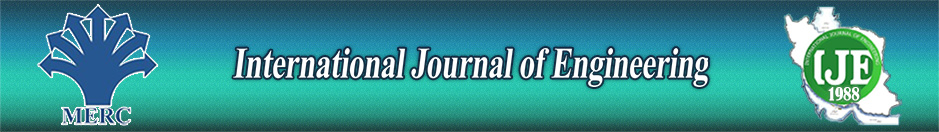Non-linear Thermo-mechanical Bending Behavior of Thin and Moderately Thick Functionally Graded Sector Plates Using Dynamic Relaxation Method

Authors

1 Mechanical Eng., Ferdowsi Univ.

2 Mechanical Eng., Islamic Azad Univ.

Abstract

In this study, nonlinear bending of solid and annular functionally graded (FG) sector plates subjected to transverse mechanical loading and thermal gradient along the thickness direction is investigated. Material properties are varied continuously along the plate thickness according to power-law distribution of the volume fraction of the constituents. According to von-Karman relation for large deflections, the two set of highly coupled nonlinear equilibrium equations are derived based on both first order shear deformation theory (FSDT) and classical plate theory (CPT). The dynamic relaxation (DR) method in conjunction with the finite difference discretization technique is used to solve the nonlinear equilibrium equations. To demonstrate the efficiency and accuracy of the present solution, some comparison studies are carried out. Effects of material grading index, boundary conditions, sector angles, thickness-to-radius ratio and thermal gradient are studied in detail. Also, to consider the effect of shear deformation and nonlinearity on the results, some linear and nonlinear analyses are carried out based on both CPT and FSDT for different thickness-to-radius ratios and boundary conditions.

Keywords